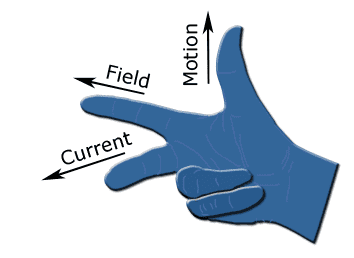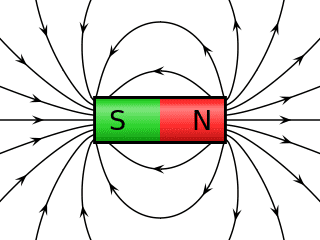# Why does Fleming's right hand rule apply for induced current

• YourBoy

#### YourBoy

I know that the right hand rule applies for induced currents, and the left hand rule is for motors, but why? It doesn't seem to connect with any other physics laws and is very counter-intuitive that the current will flow in one direction rather than the opposite direction if both are perpendicular to the path of motion and electric field, and the electric fields are symmetrical lengthwise.
Fleming's right hand rule shows that if you have a force in a certain direction and a magnetic field to in another direction, current will flow in a direction perpendicular to both. If you had a motion upwards, and a field to the left, current would flow towards you. but why not away from you? If it flows away from you it would still be perpendicular to both motion and magnetic field, so what makes it flow towards you instead of away from you?

I am looking for a physical explanation more than a mathematical one.#### Attachments

If you had a motion upwards, and a field to the left, current would flow towards you. but why not away from you?
This is a simple matter of convention. We arbitrarily define the direction of the magnetic field to point in the direction that the right hand rule works. We could have arbitrarily chosen to use a left hand rule instead. Then the direction for the field would be chosen with the opposite sense such that a left hand rule would work.

Okay, but then current would still be choosing one path over the other, why does it move in one direction particularly? The magnetic field is symmetrical lengthwise so it seems that current would not travel in either direction because the forces causing it to move would cancel out due to the field being symmetrical#### Attachments

I am looking for a physical explanation more than a mathematical one.
This is such a common request about many aspects of Physics and it is basically asking too much of 'verbal' language. If I asked you for a "Physical Reason" why and how the money that you are paying on your credit card bill is what it is then you would, I think, realize that it's all about the interest rates and the small print about what proportion gets used for this and that. You would acknowledge that it's all a matter of Maths and that it never involves actual notes and coins. Maths describes what goes on in your account and nothing else does it half as well. Why do you use a calculator? Because words don't do the job that you want.
Physics is the same only moreso. For centuries, Scientists developed the optimum way to describe the Physical World and that involves Maths. We have not found a better way to model the universe than Maths (yet). If something else turns up you can be sure it still won't involve any of the simple 'mechanical' descriptions that people crave. It will be harder than Maths, even.

•YourBoy
Okay, but then current would still be choosing one path over the other, why does it move in one direction particularly? The magnetic field is symmetrical lengthwise so it seems that current would not travel in either direction because the forces causing it to move would cancel out due to the field being symmetrical
I am not exactly sure what you are asking here. If you simply put a loop of wire around a magnet then you are correct, there is no current flow. On the other hand, if you run current through a loop of wire around a magnet (e.g. drive the current with a battery), then there will be a force exerted on that loop of wire.

why does it move in one direction particularly
Firstly, it would need to be consistent ; it would need to do the same thing every time under the same circumstances. Secondly, if we had chosen to define the direction of the field or the direction of the current, we would be using the Left Hand Rule to predict the direction of the force. That direction depends on two totally arbitrary choices of direction of two other quantities.
Edit: I was so involved with making the point that the actual direction depends on the arbitrary original choice of current and field definitions that I switched to the Left Hand Motor Rule and not the Right Hand 'Gene-RIGHT-er' Rule.

Last edited:
It looks like there is some confusion in the use of the words. In the picture, the thumb is showing something called motion. If that is the direction of the force on a current in a magnetic field, that picture is wrong, as sophiecentaur points out.

Okay, but then current would still be choosing one path over the other, why does it move in one direction particularly? The magnetic field is symmetrical lengthwise so it seems that current would not travel in either direction because the forces causing it to move would cancel out due to the field being symmetrical

Let's say a large positively and uniformly charged plate is moving to the right. The electric field lines of the plate look like this: | | | ,
and those lines are moving to the right with the plate.

An observer below the plate observes that kind of lines as depicted above. Now lets' say that that observer accelerates towards the plate. After the acceleration the lines appear to be oriented like this: / / / , according to the observer.

If those lines that I drew were sticks, the sticks would tilt the same way. When the observer accelerates, clocks at the upper ends of the sticks run faster than clocks at the lower ends of the sticks, and the upper ends move faster that the lower ends, according to the observer - that's just simple relativity.

Thats' the explanation, I think. Tilting of electric field lines.

(For more magnetic field and less electric field add a negatively charged plate moving to the left next to the positively charged plate moving to the right)

Last edited:
Okay, but then current would still be choosing one path over the other, why does it move in one direction particularly?
The induced emf is in that direction. If you don't want a mathematical explanation then you won't want to look at Maxwell's equations but you would then have to accept "that's the way it is" as an answer.

Last edited:
•davenn